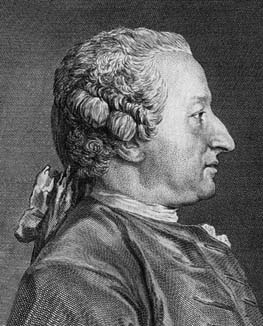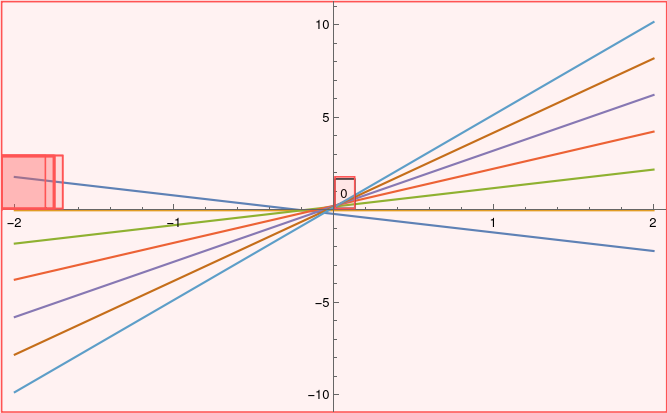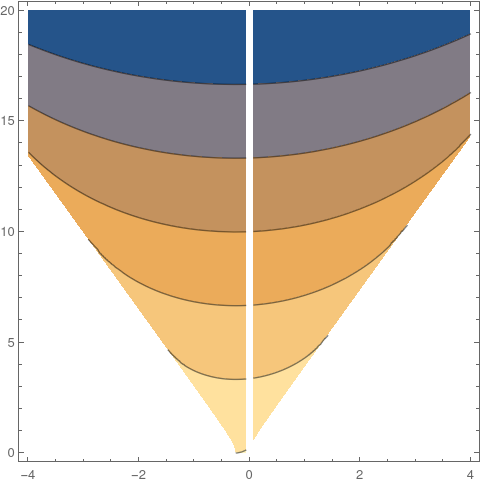# Clairaut equationsAlexis Claude Clairaut Clairaut's equation (or the Clairaut equation) is a differential equation of the form
$y(x) = x \,\frac{{\text d} y}{{\text d}x} + f \left( \frac{{\text d} y}{{\text d}x} \right) ,$
where f is continuously differentiable function. It is named after the French mathematician Alexis Clairaut (1713--1765), who introduced it in 1734.

To solve Clairaut's equation, one differentiates with respect to x, yielding

$\left[ x + f' \left( \frac{{\text d} y}{{\text d}x} \right) \right] \frac{{\text d}^2 y}{{\text d}x^2} = 0 .$
Hence, either $$\displaystyle \frac{{\text d}^2 y}{{\text d}x^2} = 0$$ or $$\displaystyle x + f' \left( \frac{{\text d} y}{{\text d}x} \right) =0.$$ In the former case, the first derivative must be a constant, $$c = {\text d}y/{\text d}x .$$ Substituting this into the Clairaut's equation, one obtains the family of straight line functions given by
$y(x) = C\,x + f\left( C \right) ,$
the so-called general solution of Clairaut's equation.

The latter case,

$x + f' \left( \frac{{\text d} y}{{\text d}x} \right) = 0$
defines only one solution y(x), the so-called singular solution, whose graph is the envelope of the graphs of the general solutions. The singular solution is usually represented using parametric notation, as (x(p), y(p)), where p = dy/dx.

Example 1: With p = dy/dx, Clairaut's equation

$y = x\,y' + \frac{y'}{4 + (y')^2} \qquad\mbox{or} \qquad y = x\,p + \frac{p}{4 + p^2} \tag{1.1}$
has the general solution
$y = x\,c + \frac{c}{4 + c^2} , \tag{1.2}$
where c is an arbitrary constant.We plot a family of solutions from the general solution: fun[f_, x_, a_List, x0_, x1_, c_, yp_, al_, off_, opts : OptionsPattern[]] := With[{p = c (x0 + x1)}, Plot[Evaluate[f[x, #] & /@ a], {x, x0, x1}, Epilog -> ({Text[Framed[g[x, #]], {p, yp f[p, #]}, {0, off}], Arrow[{{p, yp f[p, #]}, {al p, f[al p, #]}}]} & /@ a), Evaluate@FilterRules[{opts}, Options[Plot]]]] g[x_, a_] := a x + a/(a^2 + 4) fun[g, x, {-1, 0, 1, 2, 3, 4, 5}, -2, 2, s, y, arrow, offset, ImageSize -> 500] A family of solutions. Mathematica code

Clairaut's equations usually have singular solutions. To determine them, we rewrite the general solution in another form:
$y \left( 4 + c^2 \right) \left( y - cx \right) = c . -((4 + 3 c^2) x) + 2 c y = 1$
Upon differentiating with respect to c gives
$-((4 + 3 c^2) x) + 2 c y = 1 .$
D[(y - c*x)*(4 + c^2), c]
-((4 + 3 c^2) x) + 2 c y
This quadratic equation can be solved:
$c = \frac{1}{3x} \left[ y \pm \sqrt{y^2 -3x - 12 x^2} \right[ . \tag{1.3}$
Solve[-((4 + 3 c^2) x) + 2 c y == 1, c]
{{c -> (y - Sqrt[-3 x - 12 x^2 + y^2])/(3 x)}, {c -> ( y + Sqrt[-3 x - 12 x^2 + y^2])/(3 x)}}
Each braanch of quadratic solution is substituting into the general solution (1.2). Mathematica helps to visialize singular solutions *the vertical line x = 0 must excluded)
c = (y - Sqrt[-3 x - 12 x^2 + y^2])/(3 x);
Simplify[c*x + c/(4 + c^2) - y]
1/3 (-2 y - Sqrt[-3 x - 12 x^2 + y^2] + ( y - Sqrt[-3 x - 12 x^2 + y^2])/( x (4 + (y - Sqrt[-3 x - 12 x^2 + y^2])^2/(9 x^2))))
ContourPlot[ -2 y - Sqrt[-3 x - 12 x^2 + y^2] + ( y - Sqrt[-3 x - 12 x^2 + y^2])/( x (4 + (y - Sqrt[-3 x - 12 x^2 + y^2])^2/(9 x^2))), {x, -4, 4}, {y, 0, 20}]
and for another branch:
c = (y + Sqrt[-3 x - 12 x^2 + y^2])/(3 x);
Simplify[c*x + c/(4 + c^2)-y]
1/3 (-2 y + Sqrt[-3 x - 12 x^2 + y^2] + ( y + Sqrt[-3 x - 12 x^2 + y^2])/( x (4 + (y + Sqrt[-3 x - 12 x^2 + y^2])^2/(9 x^2))))
ContourPlot[-2 y + Sqrt[-3 x - 12 x^2 + y^2] + ( y + Sqrt[-3 x - 12 x^2 + y^2])/( x (4 + (y + Sqrt[-3 x - 12 x^2 + y^2])^2/(9 x^2))), {x, -4, 4}, {y, 0, 20}]Implicit plot for negative branch. Implicit plot for positive branch.

■

Example 2: We consider another Clairaut's equation

$y = x\,y' + \left( y' \right)^2 .$
Differentiating with respect to x, we find
$y' = x\,y'' + y' + 2\,y'\,y'' ,$
which rearranges to
$0 = x\,y'' + 2\,y'\,y'' .$
If we divide by y'' ≠ 0, then
$2\,y' = -x \qquad \Longrightarrow \qquad y = - \frac{x^2}{4} + c,$
for some constant c of integration. As differentiating initially may have added solutions, then we now check whether these solutions are valid:
$\mbox{RHS} = x \left( - \frac{x}{2} \right) + \left( - \frac{x}{2} \right)^2 = - \frac{x^2}{4} + c = \mbox{LHS if} \quad c=0,$
which we see to be true only when c = 0.

If we return to the case when y'' = 0, then we have a linear solution y = Ax + B, for some constants A and B. If we substitute this into Clairaut’s equation, it gives

$A\,x + B = x\,A + A^2 \qquad \Longrightarrow \qquad B = A^2 ,$
and we obtain a family of solutions (called the general solution):
$y = x\,A + A^2 .$
It has the envelope (singular solution) $$\displaystyle y = - \frac{x^2}{4} .$$

If we substitute $$\displaystyle y = z - \frac{x^2}{4}$$ into Clairaut's equation, we obtain a differential equation

$z- \frac{x^2}{4} = x \left( z' - \frac{x^2}{4} \right) + \left( z' - \frac{x^2}{4} \right)^2 \qquad \Longrightarrow \qquad z = \left( z' \right)^2 .$
Solving the differential equation for z, we get its general solution depending on two arbitrary constants:
$z(x) = \begin{cases} \frac{1}{4} \left( x- a \right)^2 , & \ x \leqslant a, \\ 0 , & \ a \leqslant x \leqslant b , \\ \frac{1}{4} \left( x- b \right)^2 , & \ x \geqslant b, \end{cases}$
where 𝑎 and b are constants satisfying -∞ ≤ 𝑎 ≤ b ≤ ∞.    ■

Generalized Clairaut's equation

The differential equation
$f \left( x\,\frac{{\text d} y}{{\text d}x}-y \right) = g \left( \frac{{\text d} y}{{\text d}x} \right) ,$
where f and g are some given smooth functions, can be solved in exactly the same manner as Clairaut's equation to obtain the general solution
$f \left( x\,C-y \right) = g \left( C \right) ,$
where C is an arbitrary constant. The generalized Clairaut's equation may also have a singular solution. If it does, it can be obtained by differentiating the above equation with respect to x to obtain \begin{equation} y''\left[ f'(xy'-y)x-g'(y') \right] =0. \label{clair:c} \end{equation} If the first term in the above equation is zero, then the generalized Clairaut's equation is recovered. If the second term in the equation is zero, then two equations can be solved together to eliminate y'. The resulting equation for y=y(x) will have no arbitrary constants and so will be a singular solution.

Example 3: Consider the generalized Clairaut's equation

$(xy'-y)^2-(y')^2-1=0.$
Since this is a generalized Clairaut's equation with f(p) = p² and g(p) = p² -1, its general solution becomes
$(x\,C-y)^2 = C^2 +1 \qquad \mbox{or}\qquad y=C\,x\pm\sqrt{C^2-1} ,$
where C > 1 is an arbitrary constant.

To find the singular solution, we differentiate the given equation with respect to x to obtain \begin{equation} y''[2(xy'-2)x-2y']=0. \end{equation} If the second term is set equal to zero, then we find \begin{equation} y'=\frac{x y}{x^2-1}. \label{clair:g} \end{equation} We determine the singular solution to be % \begin{equation} x^2+y^2=1. \label{clair:h} \end{equation} Note that this equation is not derivable from the general solution for any choice of C.    ■

The Lagrange differential equation

A differential equation of type
$y = x\, f(y' ) + g(y' ) ,$
where f and g are given functions differentiable on a certain interval, is called the Lagrange equation. It is convenient to denote by p = dy/dx, the derivative of the unknown function. Then differentiating the equation $$\displaystyle y = x\,f(p) + g(p), we obtain ${\text d}y = x\,{\text d}f(p) + f(p)\,{\text d}x + {\text d}g(p) .$ Using the relation \( \displaystyle p\,{\text d}x = {\text d}y,$$ we get
$p\,{\text d}x = x\,{\text d}f(p) + f(p)\,{\text d}x + {\text d}g(p) .$
Now move fdx to the left-hand side
$\left[ p - f(p) \right] {\text d}x = x\,{\text d}f(p) + {\text d}g(p)$
and divide both sides by dp:
$\left[ p - f(p) \right] \frac{{\text d}x}{{\text d}p} = x\,\frac{{\text d}f}{{\text d}p} + \frac{{\text d}g}{{\text d}p} .$
Assuming pf(p), we obtain
$\frac{{\text d}x}{{\text d}p} = \frac{1}{p - f(p)} \left[ x\,\frac{{\text d}f}{{\text d}p} + \frac{{\text d}g}{{\text d}p} \right] .$
This is a first-order differential equation, and its solution x(p) should be achievable depending on the details of the functions f(p) and g(p). Even its singular solution may be found be setting the singular value p = f(p) in the original differential equation.

Example 4: Consider the differential equation

$y = 3p\,x + 7\,\ln p, \qquad p = {\text d}y/{\text d}x .$
With f(p) = 3p and g(p) = 5lnp, we obtain
$\frac{{\text d}x}{{\text d}p} = -\frac{1}{2p} \left[ 3x + \frac{7}{p} \right] .$
This is a first order differential equation. Its solution becomes
$x(p) = -\frac{7}{p} + \frac{C}{p^{1/2}} ,$
where C is an arbitrary constant. Inserting this expression into the given Lagrange equation, we obtain
$x(p) = 7 \left[ \ln p -3 \right] + 3\,\frac{C}{p^{1/2}} .$
The last two equations provide the parametric representation of the general solution y = y(x). A singular solution is y(x) = 0.    ■